##### Statistics For Dummies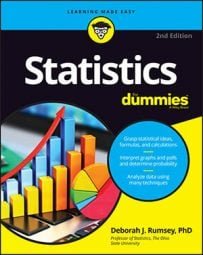In statistics, you can calculate a regression line for two variables if their scatterplot shows a linear pattern and the correlation between the variables is very strong (for example, r = 0.98). A regression line is simply a single line that best fits the data (in terms of having the smallest overall distance from the line to the points). Statisticians call this technique for finding the best-fitting line a simple linear regression analysis using the least squares method. (Phew! That's a mouthful!)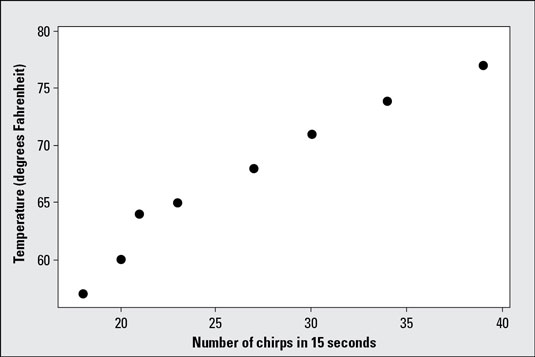Scatterplot of cricket chirps in relation to outdoor temperature.
The formula for the best-fitting line (or regression line) is y = mx + b, where m is the slope of the line and b is the y-intercept. This equation itself is the same one used to find a line in algebra; but remember, in statistics the points don’t lie perfectly on a line — the line is a model around which the data lie if a strong linear pattern exists.
• The slope of a line is the change in Y over the change in X. For example, a slope of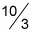means as the x-value increases (moves right) by 3 units, the y-value moves up by 10 units on average.

• The y-intercept is the value on the y-axis where the line crosses. For example, in the equation y=2x – 6, the line crosses the y-axis at the value b= –6. The coordinates of this point are (0, –6); when a line crosses the y-axis, the x-value is always 0.

You may be thinking that you have to try lots and lots of different lines to see which one fits best. Fortunately, you have a more straightforward option (although eyeballing a line on the scatterplot does help you think about what you’d expect the answer to be). The best-fitting line has a distinct slope and y-intercept that can be calculated using formulas (and these formulas aren’t too hard to calculate).

To save a great deal of time calculating the best fitting line, first find the “big five,” five summary statistics that you’ll need in your calculations:

1. The mean of the x values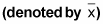2. The mean of the y values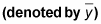3. The standard deviation of the x values (denoted sx)

4. The standard deviation of the y values (denoted sy)

5. The correlation between X and Y (denoted r)

## Finding the slope of a regression line

The formula for the slope, m, of the best-fitting line is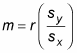where r is the correlation between X and Y, and sx and sy are the standard deviations of the x-values and the y-values, respectively. You simply divide sy by sx and multiply the result by r.

Note that the slope of the best-fitting line can be a negative number because the correlation can be a negative number. A negative slope indicates that the line is going downhill. For example, if an increase in community center programs is related to a decrease in the number of crimes in a linear fashion; then the correlation and hence the slope of the best-fitting line is negative in this case.

The correlation and the slope of the best-fitting line are not the same. The formula for slope takes the correlation (a unitless measurement) and attaches units to it. Think of sy divided by sx as the variation (resembling change) in Y over the variation in X, in units of X and Y. For example, variation in temperature (degrees Fahrenheit) over the variation in number of cricket chirps (in 15 seconds).

## Finding the y-intercept of a regression line

The formula for the y-intercept, b, of the best-fitting line is b = y̅ -mx̅, where and are the means of the x-values and the y-values, respectively, and m is the slope.

So to calculate the y-intercept, b, of the best-fitting line, you start by finding the slope, m, of the best-fitting line using the above steps. Then to find the y-intercept, you multiply m by and subtract your result from .

Always calculate the slope before the y-intercept. The formula for the y-intercept contains the slope!